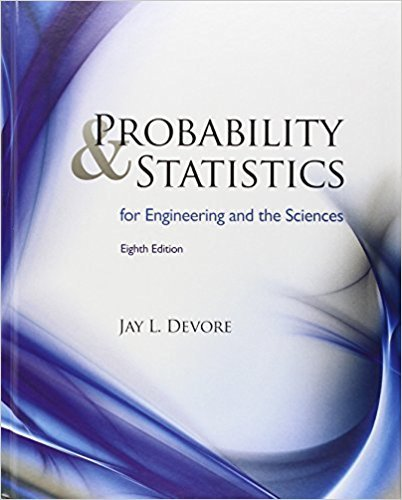×
Get Full Access to Probability And Statistics For Engineering And The Sciences - 8 Edition - Chapter 1 - Problem 18e
Get Full Access to Probability And Statistics For Engineering And The Sciences - 8 Edition - Chapter 1 - Problem 18e

×

# In a study of author productivity (“Lotka’s Test,”ISBN: 9780538733526 61

## Solution for problem 18E Chapter 1

Probability and Statistics for Engineering and the Sciences | 8th Edition

• Textbook Solutions
• 2901 Step-by-step solutions solved by professors and subject experts
• Get 24/7 help from StudySoup virtual teaching assistantsProbability and Statistics for Engineering and the Sciences | 8th Edition

4 5 1 238 Reviews
23
3
Problem 18E

Problem 18E

In a study of author productivity (“Lotka’s Test,” Collection Mgmt., 1982: 111–118), a large number of authors were classified according to the number of articles they had published during a certain period. The results were presented in the accompanying frequency distribution:a. Construct a histogram corresponding to this frequency distribution. What is the most interesting feature of the shape of the distribution?

b. What proportion of these authors published at least five papers? At least ten papers? More than ten papers?

c. Suppose the five 15s, three 16s, and three 17s had been lumped into a single category displayed as “ ≥ 15.” Would you be able to draw a histogram? Explain.

d. Suppose that instead of the values 15, 16, and 17 being listed separately, they had been combined into a 15–17 category with frequency 11. Would you be able to draw a histogram? Explain.

Step-by-Step Solution:

Step 1 of 4

(a)

The number of articles published in a certain period is presented in the accompanying frequency distribution.

 Number of papers Frequency 1 784 2 204 3 127 4 50 5 33 6 28 7 19 8 19 9 6 10 7 11 6 12 7 13 4 14 4 15 5 16 3 17 3

We are asked to construct a histogram corresponding to this frequency distribution. What is the most interesting feature of the shape of the distribution?

Step to create the Histogram in Minitab:

1. On the opening of Minitab, you will get session handler and worksheet like Excel worksheet.
2. Enter the data into the worksheet (in this case Number of papers and Frequency).
3. After entering the data go to a menu called Graph in menu panel.
4. In the Graph menu select option Histogram, you will get one window of Histogram.
5. Under the Histogram window,  and select the simple graph option.
6. After doing this, you will get another which will contain all the variables you have entered in the worksheet.
7. Select the Number of papers variable as Graph variables.
8. Click on Data Options… and select frequency tab, under that double click the Frequency variable into the Frequency variables option.
9. After everything entered correctly, press ok and you will get the Histogram of your data.
10. Right click on the graph you have just created and select the copy graph option.Figure 1: Histogram of the data

The most interesting feature of the shape of the distribution is the heavy positive skewness of the data.

Step 2 of 4

Step 3 of 4

##### ISBN: 9780538733526

This full solution covers the following key subjects: papers, frequency, histogram, distribution, been. This expansive textbook survival guide covers 16 chapters, and 1314 solutions. The answer to “In a study of author productivity (“Lotka’s Test,” Collection Mgmt., 1982: 111–118), a large number of authors were classified according to the number of articles they had published during a certain period. The results were presented in the accompanying frequency distribution: a. Construct a histogram corresponding to this frequency distribution. What is the most interesting feature of the shape of the distribution?b. What proportion of these authors published at least five papers? At least ten papers? More than ten papers?c. Suppose the five 15s, three 16s, and three 17s had been lumped into a single category displayed as “ ? 15.” Would you be able to draw a histogram? Explain.d. Suppose that instead of the values 15, 16, and 17 being listed separately, they had been combined into a 15–17 category with frequency 11. Would you be able to draw a histogram? Explain.” is broken down into a number of easy to follow steps, and 143 words. Probability and Statistics for Engineering and the Sciences was written by and is associated to the ISBN: 9780538733526. The full step-by-step solution to problem: 18E from chapter: 1 was answered by , our top Statistics solution expert on 08/08/17, 06:52AM. This textbook survival guide was created for the textbook: Probability and Statistics for Engineering and the Sciences , edition: 8. Since the solution to 18E from 1 chapter was answered, more than 2029 students have viewed the full step-by-step answer.

Unlock Textbook Solution